﻿ 3.2 Yield to Maturityoffice (412) 967-9367
fax (412) 967-5958
toll-free 1 (800) 214-3480

9.2  Constant Continuous Dividend Yield OPM

 T

he Black-Scholes option pricing model assumes that the underlying asset pays zero dividends over the life of the option.  In practice this is rarely the case.  A useful extension of the Black-Scholes model addresses an underlying asset that pays a dividend continuously with a known yield.  This assumption allows us to apply the model to a wide range of options, including currency options and options on futures. Recall that in Chapter 6, topic 6.5, Interpretation of the Black-Scholes Model, you saw that the Black-Scholes option pricing model consists of two terms.  The first term is an expected present value of the stock price, conditional upon the terminal stock price exceeding the strike price (i.e., finishing in-the-money).  The second term is the expected present  value of the strike price, also conditional on the option being in-the-money.

Black and Scholes approach the problem by assuming a well-behaved dynamic stock price process, in which the option value is a function of the underlying stock price and time.  From this assumption, a partial differential equation (PDE) that includes the unknown option valuation function and some of its derivatives is derived from an instantaneously riskless portfolio.  Finally, they solve this differential equation subject to the appropriate terminal option values to get a closed-form solution for the option value.

From the binomial option pricing model, you should have a good understanding of how the principle of dynamic replication maintains the riskless hedge over the life of the option.  Whenever this principle is applicable, there is an easier approach for valuing options, namely, the Risk-Neutral Valuation Principle (see Chapter 2, topic 2.7).  Application of this principle yields the expected present value with respect to a risk-neutral probability distribution.

Although the constant continuous dividend extension to the Black-Scholes pricing model can be derived formally from partial differential equations, the risk-neutral valuation principle provides a more intuitive approach.  It lets us view the value of a continuous dividend-paying stock as equaling the sum of two streams:  the present value of the continuous dividend plus the present value of the stock that is stripped of its dividend.

The former has no impact upon the value of the option.  Instead it is the terminal distribution of the latter that determines the value of the option.

Approach

Suppose you hold one share of security S that pays a constant continuous dividend yield q.  Changes in the value of your holding arise from two components --- realized capital changes, and dividends.  Over a small interval of time  Dt, denote the realized capital gain/loss as  DS and the dividend as qSDt.

The current stock price for this security, S, reflects both the present value of capital gains/losses plus the present value of dividends.  If the dividends were retained by the stock, then the "cum" dividend stock price at any future time, T, is larger than the actual terminal ex-dividend stock price, ST, by: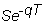As a notational note, the present is denoted as 0.  Therefore, the interval of time from the present to time T is T - 0 = T.  In terms of present values, the present value of the stock stripped of all claims to future dividends is:The terminal distribution of this stripped stock value is the underlying asset that the optionholder has a claim against at the time of the option's maturity.  As a result, the standard Black-Scholes option pricing model applies to the present value of this stripped stock.

Option Pricing Model for the Stripped Stock

Denote S' as: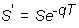Applying the standard option pricing model to S' yields: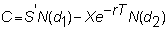where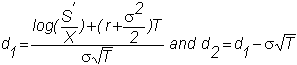Substituting the stripped stock price for S' gives the constant dividend yield option pricing model: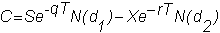and since ln(S'/X) = ln(S/X) - qT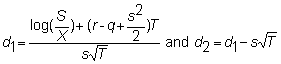Similarly, the European put option price is either obtained indirectly from put-call parity or directly expressed as: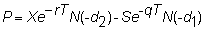where d1  and d2  are as before.

EXAMPLE

One application of the constant dividend yield model is to value options on stock indices.  A stock index such as the S&P500 is a value-weighted index of 500 stocks.  This means that the index is calculated as follows: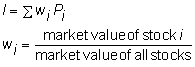Since an index such as the S&P500 contains a large number of stocks which pay dividends at different times, it is sometimes held that the constant dividend yield model is a good approximation for valuing options on this index.  The S&P500 index options also have the simpler feature of being European style options, so the option pricing formula developed in this chapter can be applied without modification.

Consider the following data.  On September 29, 1993, the value of the S&P500 index at 1:45 pm was 460.38.  The last traded prices on the October options (maturing on October 15) are:

 Strike Call Put 455 7 7/8 2 1/4 460 4 3/8 3 7/8 465 1 7/8 6 3/8

The interest rate on a Treasury bill maturing on October 14 was 2.835.  Here,

S = 460.38

T = 0.0438

r = 0.02835

The dividend yield on the S&P500 index is estimated at 2%, so q = 0.02.  We will use the volatility estimate implicit in the at-the-money option prices.  You can do this using the Option Calculator subject of Option Tutor as follows.

First, select Call Option, then European.  Now, select “On” for implied volatility.  Enter 4 3/8 = 4.375 for the Option Price.  Put in the numbers for the asset price (460.38), the maturity (0.0438), the interest rate (0.02835), and the dividend yield (0.02).  Enter 460 for the strike price, and click OK.

The implied volatility (or sigma) is 0.1067.

Now, enter this value for the volatility, and select “Off” for implied volatility.  Now, we can use this volatility estimate to value the other options.   This yields the following put and call prices, compared to the actual market prices.

 Actual Estimated Strike Call Put Call Put 455 7 7/8 2 1/4 7.430 1.887 460 4 3/8 3 7/8 4.375 3.827 465 1 7/8 6 3/8 2.270 6.716

You can see that applying this model with a constant volatility estimate, undervalues the 455 strike options and overvalues the 465 call.  Such a systematic effect is often referred to as the "volatility smile."

The constant dividend yield  model  provides a useful model for pricing a wide variety of derivatives such as currency options and options on futures.  It is therefore useful for a wide range of risk management problems.  We provide the comparative statics for this model in the next topic,  Comparative Statics.